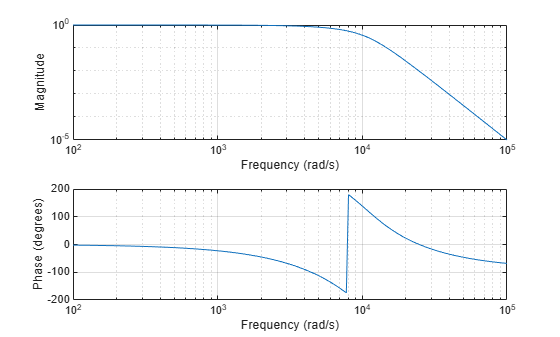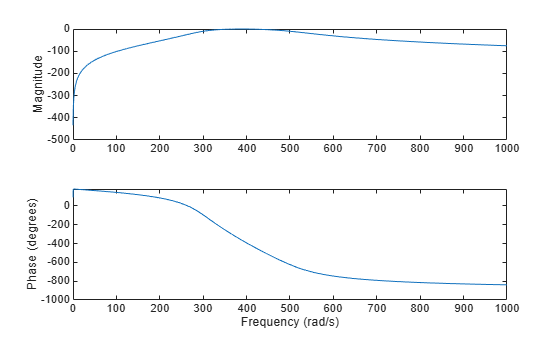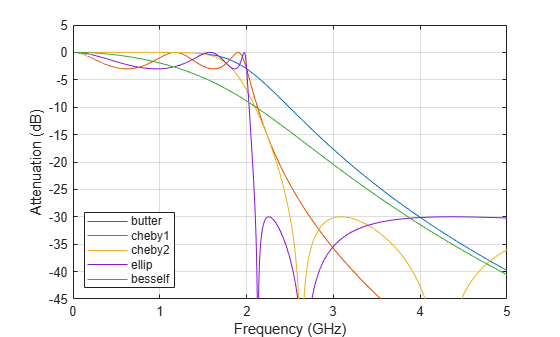# besself

Bessel analog filter design

## Syntax

``[b,a] = besself(n,Wo)``
``[b,a] = besself(n,Wo,ftype)``
``[z,p,k] = besself(___)``
``[A,B,C,D] = besself(___)``

## Description

example

````[b,a] = besself(n,Wo)` returns the transfer function coefficients of an `n`th-order lowpass analog Bessel filter, where `Wo` is the angular frequency up to which the filter's group delay is approximately constant. Larger values of `n` produce a group delay that better approximates a constant up to `Wo`. The `besself` function does not support the design of digital Bessel filters.```

example

````[b,a] = besself(n,Wo,ftype)` designs a lowpass, highpass, bandpass, or bandstop analog Bessel filter, depending on the value of `ftype` and the number of elements of `Wo`. The resulting bandpass and bandstop designs are of order 2`n`.```

example

````[z,p,k] = besself(___)` designs a lowpass, highpass, bandpass, or bandstop analog Bessel filter and returns its zeros, poles, and gain. This syntax can include any of the input arguments in previous syntaxes.```
````[A,B,C,D] = besself(___)` designs a lowpass, highpass, bandpass, or bandstop analog Bessel filter and returns the matrices that specify its state-space representation.```

## Examples

collapse all

Design a fifth-order analog lowpass Bessel filter with approximately constant group delay up to $1{0}^{4}$ rad/second. Plot the magnitude and phase responses of the filter using `freqs`.

```[b,a] = besself(5,10000); freqs(b,a)```Compute the group delay response of the filter as the derivative of the unwrapped phase response. Plot the group delay to verify that it is approximately constant up to the cutoff frequency.

```[h,w] = freqs(b,a,1000); grpdel = diff(unwrap(angle(h)))./diff(w); clf semilogx(w(2:end),grpdel) xlabel('Frequency (rad/s)') ylabel('Group delay (s)')```Design a 12th-order bandpass Bessel filter with the passband ranging from 300 rad/s to 500 rad/s. Compute the frequency response of the filter.

```[b,a] = besself(6,[300 500],'bandpass'); [h,w] = freqs(b,a);```

Plot the magnitude and phase responses of the filter. Unwrap the phase response to avoid ${180}^{\circ }$ and ${360}^{\circ }$ jumps and convert it from radians to degrees. As expected, the phase response is close to linear over the passband.

```subplot(2,1,1) plot(w,20*log10(abs(h))) ylabel('Magnitude') subplot(2,1,2) plot(w,180*unwrap(angle(h))/pi) ylabel('Phase (degrees)') xlabel('Frequency (rad/s)')```Design a 5th-order analog Butterworth lowpass filter with a cutoff frequency of 2 GHz. Multiply by $2\pi$ to convert the frequency to radians per second. Compute the frequency response of the filter at 4096 points.

```n = 5; fc = 2e9; [zb,pb,kb] = butter(n,2*pi*fc,"s"); [bb,ab] = zp2tf(zb,pb,kb); [hb,wb] = freqs(bb,ab,4096);```

Design a 5th-order Chebyshev Type I filter with the same edge frequency and 3 dB of passband ripple. Compute its frequency response.

```[z1,p1,k1] = cheby1(n,3,2*pi*fc,"s"); [b1,a1] = zp2tf(z1,p1,k1); [h1,w1] = freqs(b1,a1,4096);```

Design a 5th-order Chebyshev Type II filter with the same edge frequency and 30 dB of stopband attenuation. Compute its frequency response.

```[z2,p2,k2] = cheby2(n,30,2*pi*fc,"s"); [b2,a2] = zp2tf(z2,p2,k2); [h2,w2] = freqs(b2,a2,4096);```

Design a 5th-order elliptic filter with the same edge frequency, 3 dB of passband ripple, and 30 dB of stopband attenuation. Compute its frequency response.

```[ze,pe,ke] = ellip(n,3,30,2*pi*fc,"s"); [be,ae] = zp2tf(ze,pe,ke); [he,we] = freqs(be,ae,4096);```

Design a 5th-order Bessel filter with the same edge frequency. Compute its frequency response.

```[zf,pf,kf] = besself(n,2*pi*fc); [bf,af] = zp2tf(zf,pf,kf); [hf,wf] = freqs(bf,af,4096);```

Plot the attenuation in decibels. Express the frequency in gigahertz. Compare the filters.

```plot([wb w1 w2 we wf]/(2e9*pi), ... mag2db(abs([hb h1 h2 he hf]))) axis([0 5 -45 5]) grid xlabel("Frequency (GHz)") ylabel("Attenuation (dB)") legend(["butter" "cheby1" "cheby2" "ellip" "besself"])```The Butterworth and Chebyshev Type II filters have flat passbands and wide transition bands. The Chebyshev Type I and elliptic filters roll off faster but have passband ripple. The frequency input to the Chebyshev Type II design function sets the beginning of the stopband rather than the end of the passband. The Bessel filter has approximately constant group delay along the passband.

## Input Arguments

collapse all

Filter order, specified as an integer scalar. For bandpass and bandstop designs, `n` represents one-half the filter order.

Data Types: `double`

Cutoff frequency, specified as a scalar or a two-element vector. A cutoff frequency is an upper or lower bound of the frequency range in which the filter's group delay is approximately constant. Cutoff frequencies must be expressed in radians per second and can take on any positive value.

• If `Wo` is scalar, then `besself` designs a lowpass or highpass filter with cutoff frequency `Wo`.

• If `Wo` is a two-element vector `[w1 w2]`, where `w1` < `w2`, then `besself` designs a bandpass or bandstop filter with lower cutoff frequency `w1` and higher cutoff frequency `w2`.

Data Types: `double`

Filter type, specified as:

• `'low'` — a lowpass filter with cutoff frequency `Wo`. `'low'` is the default for scalar `Wo`.

• `'high'` — a highpass filter with cutoff frequency `Wo`.

• `'bandpass'` — a bandpass filter of order 2`n` if `Wo` is a two-element vector. `'bandpass'` is the default when `Wo` has two elements.

• `'stop'` — a bandstop filter of order 2`n` if `Wo` is a two-element vector.

## Output Arguments

collapse all

Transfer function coefficients of the filter, returned as row vectors of length `n` + 1 for lowpass and highpass filters and 2`n` + 1 for bandpass and bandstop filters. The transfer function is expressed in terms of `b` and `a` as

`$H\left(s\right)=\frac{B\left(s\right)}{A\left(s\right)}=\frac{\text{b(1)}\text{\hspace{0.17em}}{s}^{n}+\text{b(2)}\text{\hspace{0.17em}}{s}^{n-1}+\cdots +\text{b(n+1)}}{\text{a(1)}\text{\hspace{0.17em}}{s}^{n}+\text{a(2)}\text{\hspace{0.17em}}{s}^{n-1}+\cdots +\text{a(n+1)}}.$`

Data Types: `double`

Zeros, poles, and gain of the filter, returned as two column vectors of length `n` (2`n` for bandpass and bandstop designs) and a scalar. The transfer function is expressed in terms of `z`, `p`, and `k` as

`$H\left(s\right)=\text{k}\frac{\left(s-\text{z(1)}\right)\text{\hspace{0.17em}}\left(s-\text{z(2)}\right)\cdots \left(s-\text{z(n)}\right)}{\left(s-\text{p(1)}\right)\text{\hspace{0.17em}}\left(s-\text{p(2)}\right)\cdots \left(s-\text{p(n)}\right)}.$`

Data Types: `double`

State-space representation of the filter, returned as matrices. If m = `n` for lowpass and highpass designs and m = 2`n` for bandpass and bandstop filters, then `A` is m × m, `B` is m × 1, `C` is 1 × m, and `D` is 1 × 1.

The state-space matrices relate the state vector x, the input u, and the output y through

`$\begin{array}{l}\stackrel{˙}{x}=\text{A}\text{\hspace{0.17em}}x+\text{B}\text{\hspace{0.17em}}u\\ y=\text{C}\text{\hspace{0.17em}}x+\text{D}\text{\hspace{0.17em}}u.\end{array}$`

Data Types: `double`

## Algorithms

`besself` designs analog Bessel filters, which are characterized by an almost constant group delay across the entire passband, thus preserving the wave shape of filtered signals in the passband.

Lowpass Bessel filters have a monotonically decreasing magnitude response, as do lowpass Butterworth filters. Compared to the Butterworth, Chebyshev, and elliptic filters, the Bessel filter has the slowest rolloff and requires the highest order to meet an attenuation specification.

For high-order filters, the state-space form is the most numerically accurate, followed by the zero-pole-gain form. The transfer function coefficient form is the least accurate; numerical problems can arise for filter orders as low as 15.

`besself` uses a four-step algorithm:

1. Find lowpass analog prototype poles, zeros, and gain using the `besselap` function.

2. Convert the poles, zeros, and gain into state-space form.

3. If required, use a state-space transformation to convert the lowpass filter into a bandpass, highpass, or bandstop filter with the desired frequency constraints.

4. Convert the state-space filter back to transfer function or zero-pole-gain form, as required.

 Parks, Thomas W., and C. Sidney Burrus. Digital Filter Design. New York: John Wiley & Sons, 1987.